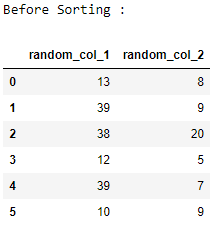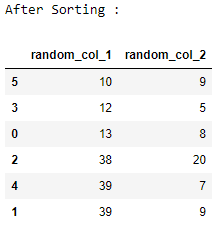Open In App

# Generating Random Integers in Pandas Dataframe

Pandas is the most popular Python library that is used for data analysis. It provides highly optimized performance with back-end source code that is purely written in C or Python. Here we will see how to generate random integers in the Pandas datagram. We will be using the numpy.random.randint() method to generate random integers.

## Generating random integers under a Single Data frame column

Generating 11 random integers from 5 to 35.

## python3

 `# importing pandas and numpy libraries``import` `numpy as np``import` `pandas as pd` `data ``=` `np.random.randint(``5``, ``35``, size``=``11``)``df ``=` `pd.DataFrame(data, columns``=``[``'random_numbers'``])` `# displaying random integers in data frame``print``(df)`

Output :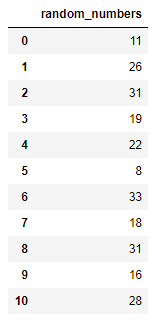## Sorting a column under the Single Data frame column

Sorting the random integer values using dataframe.sort_values() and displaying them.

## python3

 `# importing pandas and numpy libraries``import` `numpy as np``import` `pandas as pd` `# generating 7 random integers from 5 to 35``data ``=` `np.random.randint(``5``, ``35``, size ``=` `7``)``df ``=` `pd.DataFrame(data, columns ``=` `[``'integers'``])` `# displaying random integers in data frame``print``(``"Before Sorting :"``)``print``(df)` `df.sort_values(``"integers"``, axis ``=` `0``, ascending ``=` `True``,``                ``inplace ``=` `True``, na_position ``=``'last'``)` `print``(``"After Sorting :"``)``print``(df)`

Output :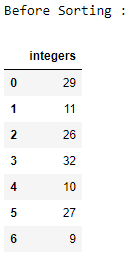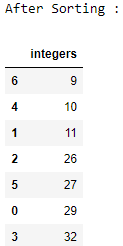## Generating random integers under the Multiple Data frame column

Generating 12X3 i.e 36 random integers from 5 to 40.

## python3

 `# importing pandas and numpy libraries``import` `numpy as np``import` `pandas as pd` `data ``=` `np.random.randint(``5``, ``40``, size``=``(``12``, ``3``))``df ``=` `pd.DataFrame(data, columns``=``[``'random_no_1'``,``                                 ``'random_no_2'``,``                                 ``'random_no_3'``])` `# displaying random integers in the dataframe``print``(df)`

Output :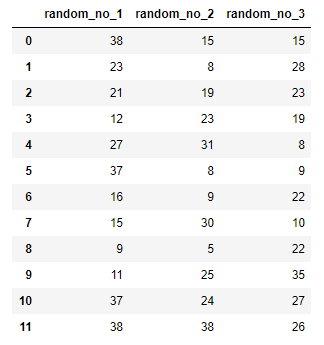## Sorting random integers columns in Pandas Multiple Data frame column

Sorting both Random integer columns, First column 1 is sorted then for every column 1, column 2 is sorted in ascending order using dataframe.sort_values().

## python3

 `# importing pandas and numpy libraries``import` `numpy as np``import` `pandas as pd` `# generating 6x2 i.e 12 random integers``# from 5 to 40``data ``=` `np.random.randint(``5``, ``40``, size ``=` `(``6``, ``2``))``df ``=` `pd.DataFrame(data, columns ``=` `[``'random_col_1'``, ``'random_col_2'``])` `# displaying random integers in data frame``print``(``"Before Sorting :"``)``print``(df)` `df.sort_values([``'random_col_1'``, ``'random_col_2'``], axis ``=` `0``,``            ``ascending ``=` `[``True``, ``True``], inplace ``=` `True``)` `print``(``"After Sorting :"``)``print``(df)`

Output :